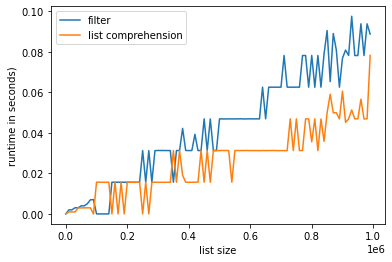# Difference between List comprehension and Lambda in Python

List comprehension is an elegant way to define and create a list in Python. We can create lists just like mathematical statements and in one line only. The syntax of list comprehension is easier to grasp.

A list comprehension generally consists of these parts :

• Output expression,
• Input sequence,
• A variable representing a member of the input sequence and
• An optional predicate part.

### Syntax of list comprehension

`List = [expression(i) for i in another_list if filter(i)]`

Example:

## Python3

 `lst ``=` `[x ``*``*` `2` `for` `x ``in` `range``(``1``, ``11``) ``if` `x ``%` `2` `=``=` `1``]` `print``(lst)`

Output:

`[1, 9, 25, 49, 81]`

In the above example,

• x ** 2 is the expression.
• range (1, 11) is an input sequence or another list.
• x is the variable.
• if x % 2 == 1 is predicate part.

## What is lambda?

In Python, an anonymous function means that a function is without a name. As we already know the def keyword is used to define the normal functions and the lambda keyword is used to create anonymous functions. It has the following syntax:

### Syntax of lambda

`lambda arguments : expression`

Example:

## Python3

 `lst ``=` `list``(``map``(``lambda` `x: x``*``*``2``, ``range``(``1``, ``5``)))` `print``(lst)`

Output:

`[1, 4, 9, 16]`

## The difference between Lambda and List Comprehension

List Comprehension is used to create lists, Lambda is function that can process like other functions and thus return values or lists.

Example:

## Python3

 `# list from range 0 to 10` `list_ ``=` `[``0``, ``1``, ``2``, ``3``, ``4``, ``5``, ``6``, ``7``, ``8``, ``9``]` `print``(list_)` ` `  `# lambda function` `lambda_list ``=` `list``(``map``(``lambda` `x: x ``*` `2``, list_))`   `# Map basically iterates every element` `# in the list_ and returns the lambda ` `# function result` `print``(lambda_list)` ` `  `# list comprehension` `list_comp ``=` `[x ``*` `2` `for` `x ``in` `list_]` `print``(list_comp)`

Output:

```[0, 1, 2, 3, 4, 5, 6, 7, 8, 9]
[0, 2, 4, 6, 8, 10, 12, 14, 16, 18]
[0, 2, 4, 6, 8, 10, 12, 14, 16, 18]```

## Graphical representation of list comprehension vs lambda + filter

As we can see from the graph that overall list comprehension is much faster than the filter function. The filter is faster for a small list only.

## Python3

 `import` `numpy as np` `import` `matplotlib.pyplot as plt` `import` `time`     `# Compare runtime of both methods` `sizes ``=` `[i ``*` `10000` `for` `i ``in` `range``(``100``)]`   `filter_runtimes ``=` `[]` `list_comp_runtimes ``=` `[]`   `for` `lis_size ``in` `sizes:`   `    ``lst ``=` `list``(``range``(lis_size))`   `    ``# Get time stamps` `    ``time_A ``=` `time.time()` `    ``list``(``filter``(``lambda` `x: x ``%` `2``, lst))` `    ``time_B ``=` `time.time()` `    ``[x ``for` `x ``in` `lst ``if` `x ``%` `2``]` `    ``time_C ``=` `time.time()`   `    ``# Calculate runtimes` `    ``filter_runtimes.append((lis_size, time_B ``-` `time_A))` `    ``list_comp_runtimes.append((lis_size, time_C ``-` `time_B))`     `# list comprehension vs. lambda + filter using Matplotlib`   `filt ``=` `np.array(filter_runtimes)` `lis ``=` `np.array(list_comp_runtimes)`   `plt.plot(filt[:, ``0``], filt[:, ``1``], label``=``'filter'``)` `plt.plot(lis[:, ``0``], lis[:, ``1``], label``=``'list comprehension'``)`   `plt.xlabel(``'list size'``)` `plt.ylabel(``'runtime in seconds)'``)`   `plt.legend()` `plt.show()`

Output:Whether you're preparing for your first job interview or aiming to upskill in this ever-evolving tech landscape, GeeksforGeeks Courses are your key to success. We provide top-quality content at affordable prices, all geared towards accelerating your growth in a time-bound manner. Join the millions we've already empowered, and we're here to do the same for you. Don't miss out - check it out now!

Previous
Next